## Relaxation Time, Collision Time and Mean Free Path:

The relaxation time is closely related to the mean time of flight between collision and also to the mean free path of the conduction electrons, which is the average distance of undisturbed motion between collision. All the collision processes occurring in the electron gas may be explained through relaxation time. The collisions are caused by thermal or structural imperfections in the lattice. The relaxation time is introduced as the characteristic time governing the establishment of equilibrium, through collision, from an initial disturbed situation in which vx ≠ 0.

The relaxation time is mathematically understood as follows: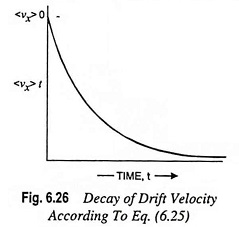Let the electrons in a metal have an average velocity <vx> 0 at any instant, t = 0; given that for t > 0 there is no external field, the electrons interact only with the lattice and ultimately the average velocity will go to zero. At any instant t(> 0), let the velocity be given by the expression (Fig. 6.26)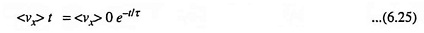where τ is the relaxation time of electrons.

The rate of change of velocity due to collision becomes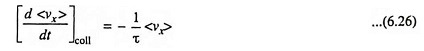The subscript “coll” refers to collisions carried out by the electrons with the lattice.

Equation (6.25) is the solution of a modified equation of motion of electrons if we consider the frictional forces due to collisions.

Considering the relaxation time, the frictional force on the electrons due to collision may be expressed as m <vx>/τ. Hence, the modified equation of electrons becomes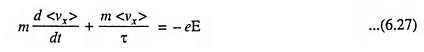In the absence of the external forces (– eE), the free motion satisfies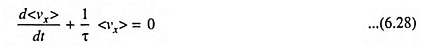Or, if <vx> 0 is the initial drift velocity in the non-equilibrium distribution, the approach to the equilibrium is described by the appropriate solution of Eq. (6.28)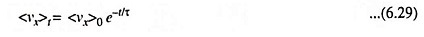The disturbances from equilibrium die out exponentially.

Let us now consider the collisions from the point of view of time. Assume that the probability for an electron to collide with a lattice (obstacle) during a short time interval dt is given by dt/τ′, where τ′ is a constant with the dimensions of time. Let F(t) represent the probability that an electron moves for a time t without suffering a collision, and let F(t + dt) represent the same probability for a period (t + dt). We may now write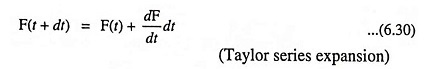and from probability theory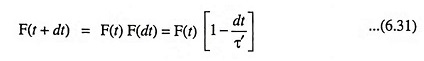Comparing Eqs. (6.30) and (6.31), we have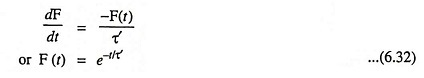Since F(t) = 1 for t = 0 which fixes the integration constant. The average time which elapses between collisions is given as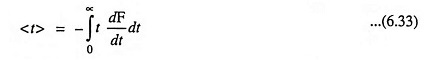because -dF/dt gives the probability that the electron collides between t and (t + dt). Substituting F(t) from Eq. (6.32) in Eq. (6.33), we have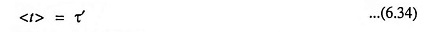Hence, the physical meaning of τ′ introduced in the proba­bilities above is that it represents the average time of collisions.

For isotropic materials, the mean time of collision is the same as the relaxation time, i.e., τ′ = τ. This is not true for non-isotropic materials.

The mean free path of an electron, λ, will be defined as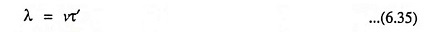where v is an appropriate average velocity of electron. In the case of metals, the conductivity always refers to a single total velocity, the one corresponding to Fermi energy. We know that free electrons in a metal can accept a series of discrete energy levels. At absolute zero, all energy levels below a certain value EF are filled, and those above EF being empty, EF is called the Fermi level of electrons. The magnitude of EF is of the order of several electron volts. At any temperature T, the probability of a state corresponding to the energy E being occupied by an electron is given as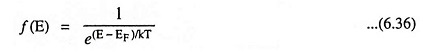where k is Boltzmann’s constant.

The velocity of an electron with Fermi energy, vF is given as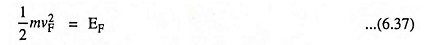Thus mean free path,

Scroll to Top